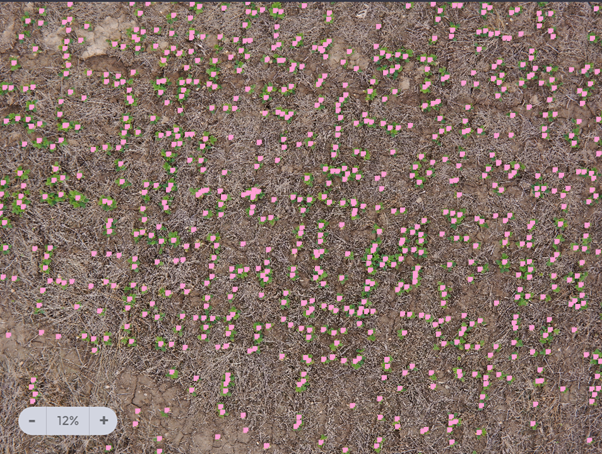# Plant Population Counting

Assessing the plants population of fields, specifically right after the emergence stage, is used for early estimation of yield.

The two common methodologies for plant population assessment in early stages are the “1 per 1000” and the “hoop” method. The “1 per 1000” method is commonly used when sawing in rows, while the “hoop” method is commonly used when there are no applicable uniform row structure in the field. Both methods are used during early scouting of the field, where the field is manually scouted in a couple of locations randomly selected in the field. The plant population estimates calculated in each of the spots are then averaged to give the general estimate of the population in the field.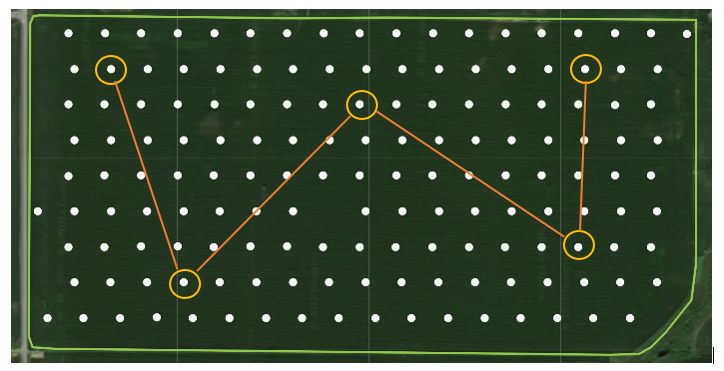The high sampling rate of the fields allows us to decrease the methods’ measuring errors (as the standard deviation of the average plant population decreases by a factor of the squared root of the number of sampling points). This means that assuming we take 10 times more samples than the traditional manual scouting, then our average population estimation error will be less than a third compared to that of the manual one. In cases where our sampling rate is as high as 100 times more than the manual scouting, then the estimation error of the plant population decreases by a factor of 10!

With Taranis’s AcreForward™ solution for plant population in early time after planting, we use our AI based solutions to scout the fields with a sampling density of over 10-100 times more than in manual scouting. Our AI based solution enables us to analyze all the images collected in these sampling points and detect all the crop plants in the image with a very high accuracy (error rate of less than 4%).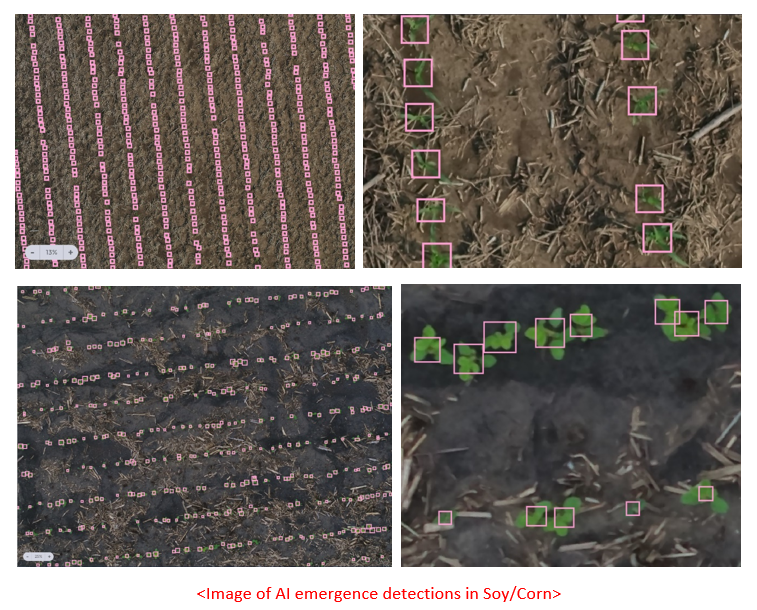Note: AcreForward™ image footprints are ranging between 100 sqft (10 sqm) for densely seeded fields and 400 sqft (37 sqm) for sparsely seeded.
For the field level average plant population, we exclude extreme values based on common statistical outlier detection methods. This gives a more robust estimation of the field’s average.

Another advantage of our high sampling rate is the ability to present the variance of the plant population across the field: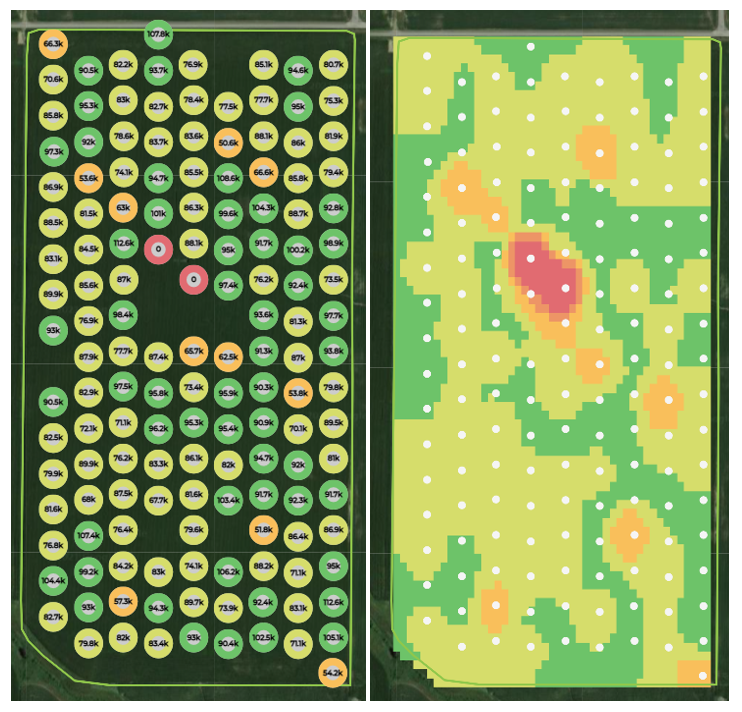To make sure the plant population calculated from the AcreForward™ images is objective and takes into account all the information gathered from the large amount of sample images, we developed an adaptation of the traditional “1 per 1000” and the “hoop” methods. These adaptations allow us to consider all the rows/plants captured, which means that even our statistics per image is more representative to that location in the field (e.g. 1/1000 uses only a single row in a given location to assess the planting density; we consider all the rows we capture in the image).

In the following section we will explain these adaptations and give some intuition as to why they are even better than the traditional methods.

# Assessing plant population using adaptive 1 per 1000

Let’s consider a simple Corn field image example. In the traditional 1/1000 it is custom to select a single row in each scout sampling location, then randomly select a segment of a predefined length inside the row and count the amount of plants in this segment. The plants count multiplied by 1000, is then the estimate of the plants density per acre in that location. The length of the segment is determined by a table based on the row spacing of the field.

To explain the intuition behind the method, let’s consider the image below to be a representative of a uniformly seeded field. Then we can imagine that this field is built out of a repeating pattern of evenly sized tiles. Each such tile is holding exactly one row in its center.

Armed with this understanding, then the reasoning behind the 1/1000 method is quite simple. Each row segment that is sampled in the field, represents an area (yes, area; not length) of 1/1000 of an acre (the 1/1000 ratio is used merely for the sake of easing the calculations). This explains why the length of the segment is defined according to the row spacing; it is actually based on the following equation: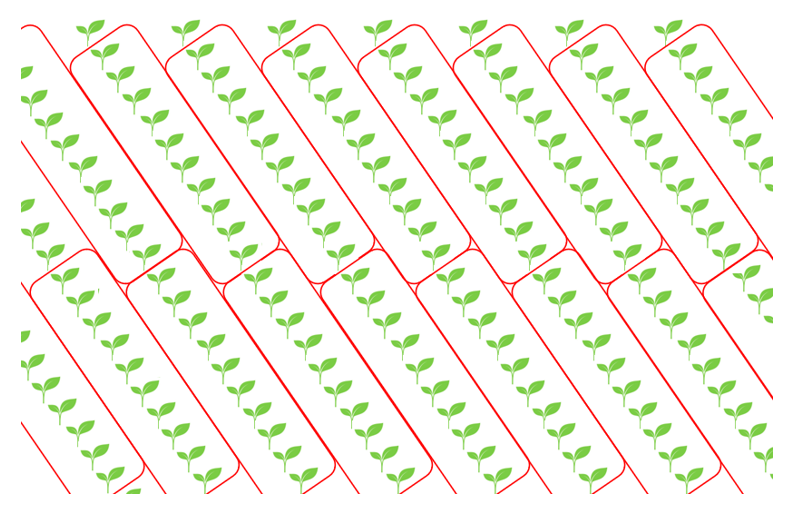Now that we have a good intuition for the math behind the “1/1000” method, we can explain the method’s modification when it comes to smart scout images.
The first generalization of the traditional method is that we can assess the row segment plants density for any row length and any row spacing by the following formula: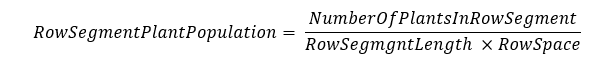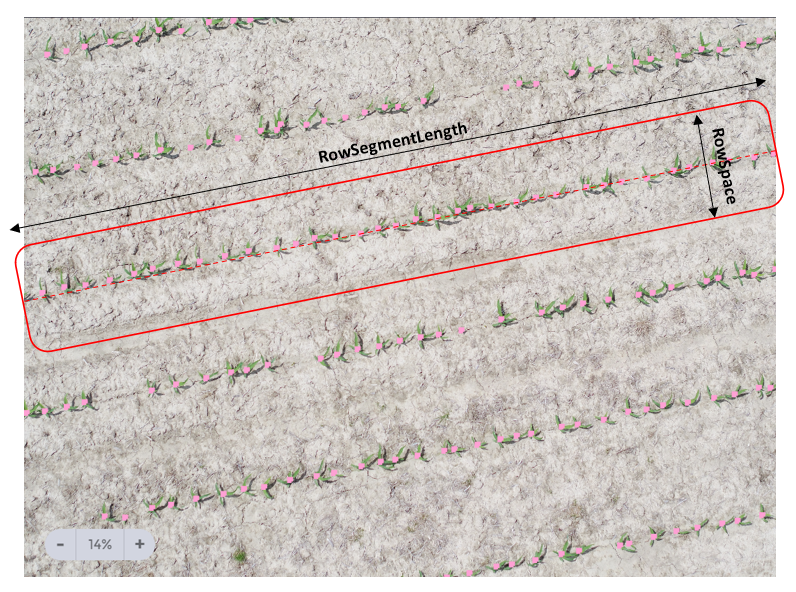This means we can get an estimate of the plant population from any row in the sample image. So now it is quite easy to infer that wanting to assess the plants density in the image, an accurate way to do so is by averaging the plant population estimate of all the captured row segments… but hey, there are long rows and very short rows in the image – how can we cope with that?
Well… the simple answer is that we use weighted average – so each row impacts the average relatively to it’s length. The longer the row – the more it impacts the measurement.

So without getting back into the math… just as an intuition – it is just as if we took all those row segments and placed them along a single line as if they were part of the same row. Now all that is left is to use the first generalization to acquire an accurate estimate for the plants density in the image.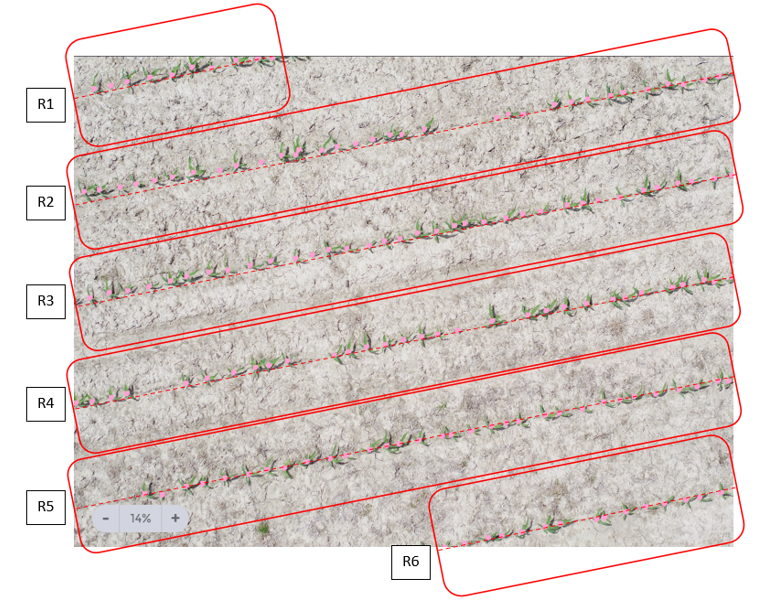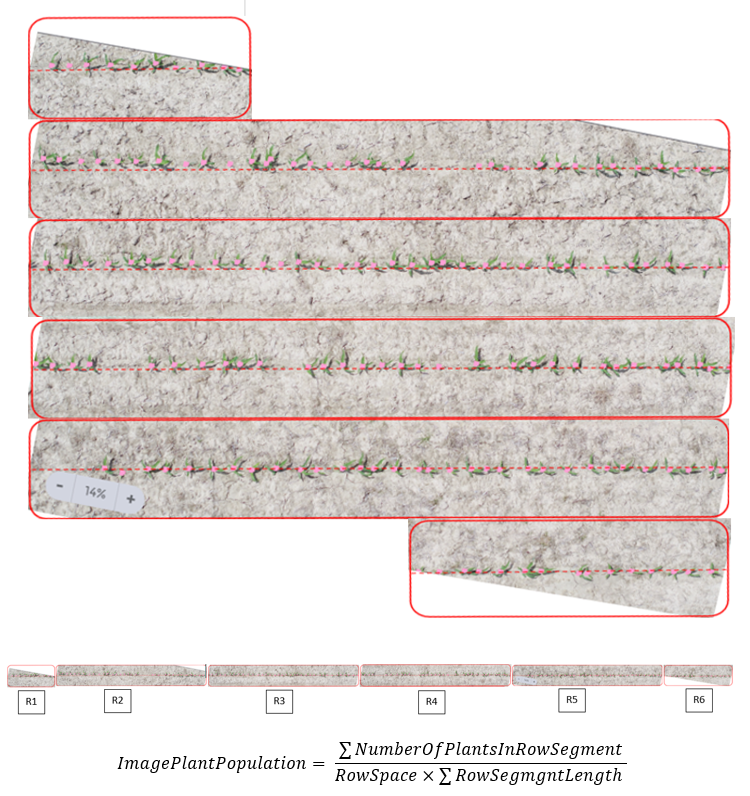Note that in fields that suffer from a high variance in the emergence or the seeds quality – the differences between the estimates based on a single row can vary a lot when just picking a neighboring row. Using our modified”1/1000” method, the estimate is more robust and indicative of the field’s status in the given location.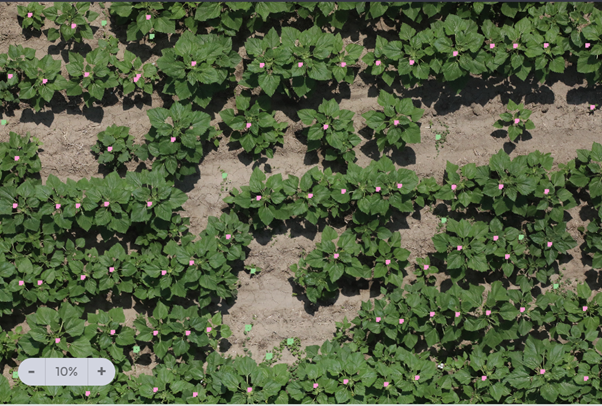# Assessing plant population using adaptive hoop

The traditional hoop method is based on measuring the plant density inside a frame or a hoop with known area. Once the density is obtained – it is merely converted to the common density measure of plants per acre.

E.g. if you use a frame with a known side length of 25 inches then its area of 4.36 sqft is equivalent to 1/10,000 of an acre. So in case there are 30 plants inside the frame, we say that the plans density is 300,000 plants per acre.

Well, in this case the intuition and generalization for using hoop with SmartScout™ images is straightforward. We just assume we have a frame covering an area equals to the footprint of the image (which can easily be calculated based on the geometry of the camera and the height above ground of the drone).

E.g. below is an image with a known ground footprint of 161 sqft in which we detected 810 Soybean plants. The plant density in the image is then calculated as follows: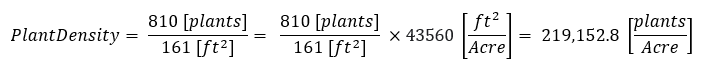In Taranis we use the “1/1000” method to calculate plant population in all cases where the field’s row structure is uniform and definitive. In the following exceptional cases, we default to the “hoop“ method calculation:

1.  No row structure in the field
2. Row structure that is not unified due to seeding geometry (e.g. tween rows), seeding problems, emergence problems or flood impact on rows structure
3. Row spacing is very small, less than 15 inch (more relevant for crops like Soybean & Rapeseed)